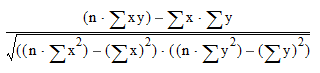# Correlation Matrix

## Teradata Warehouse Miner User Guide - Volume 1Introduction and Profiling

brand
Software
prodname
vrm_release
5.4.4
category
User Guide
featnum
B035-2300-077K

The Correlation Matrix analysis (not available in the Teradata Profiler product) allows you to build and view a Pearson Product-Moment Correlation matrix. A Pearson Product-Moment Correlation value is calculated for each pairwise combination of columns within the selected table. This is calculated as follows, for each pairwise combination of columns X and Y.where n is the total number of rows in the calculation.

The Correlation Matrix analysis must operate on numeric data. Columns of type DATE will not produce meaningful results. For NULL values, listwise deletion is automatically performed. This means that if the value of any column to be included in the matrix is NULL, the entire row is omitted during matrix calculations. Additionally, the matrix width, or the maximum number of select list items in each SQL statement, is set to 35, while the number of threads or simultaneous connections to the data source is set to 5.

Note that this does not result in a Matrix being saved in metadata for use in Export Matrix, Linear Regression and Factor Analysis. Use the Matrix Function (Matrix) for this. The Matrix function should also be used if you desire a different matrix width or number of connections.